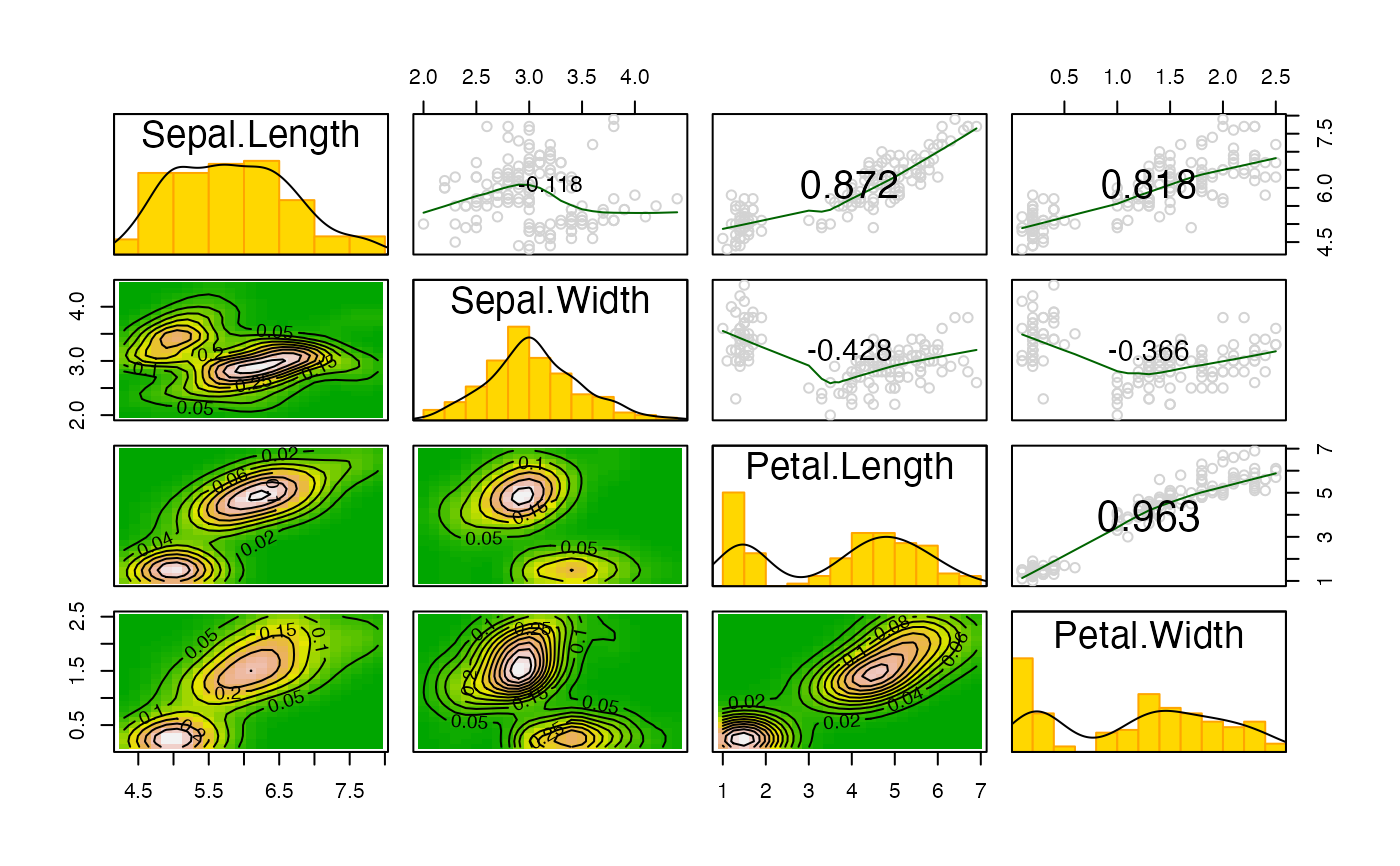Scatterplot matrix with 2D kernel density.

kdepairs(x, ...)

# S3 method for default
kdepairs(x, n=25, density=TRUE, contour=TRUE, ...)

# S3 method for rsf
kdepairs(x, n=25, density=TRUE, contour=TRUE, ...)

## Arguments

x a matrix or data frame (or a fitted model object of class "rsf" or "rspf"). number of bins to be used in kernel density estimation. logical, if shades corresponding to densities should be plotted. logical, if contour on top of shades should be plotted. other possible arguments passed to pairs.

## Value

Produces a scatterplot matrix with histograms in diagonal, 2D kernel density estimates and contours in the lower half and bivariate scatterplots with lowess smooth curves and Pearson correlation values in the upper half as a side effect. Returns NULL invisibly.

pairs, lowess, kde2d, contour
kdepairs(iris[1:4])